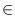Next: Minimum Cut ( min_cut Up: Graph Algorithms Previous: Maximum Flow ( max_flow   Contents   Index

# Min Cost Flow Algorithms ( min_cost_flow )

 bool FEASIBLE_FLOW(const graph& G, const node_array& supply, const edge_array& lcap, const edge_array& ucap, edge_array& flow) FEASIBLE_FLOW takes as arguments a directed graph G, two edge_arrays lcap and ucap giving for each edge a lower and upper capacity bound, an edge_array cost specifying for each edge an integer cost and a node_array supply defining for each node v a supply or demand (if supply[v] < 0). If a feasible flow (fulfilling the capacity and mass balance conditions) exists it computes such a flow and returns true, otherwise false is returned. bool FEASIBLE_FLOW(const graph& G, const node_array& supply, const edge_array& cap, edge_array& flow) as above, but assumes that lcap[e] = 0 for every edge eE. bool MIN_COST_FLOW(const graph& G, const edge_array& lcap, const edge_array& ucap, const edge_array& cost, const node_array& supply, edge_array& flow) MIN_COST_FLOW takes as arguments a directed graph G(V, E), an edge_array lcap (ucap) giving for each edge a lower (upper) capacity bound, an edge_array cost specifying for each edge an integer cost and a node_array supply defining for each node v a supply or demand (if supply[v] < 0). If a feasible flow (fulfilling the capacity and mass balance conditions) exists it computes such a flow of minimal cost and returns true, otherwise false is returned. bool MIN_COST_FLOW(const graph& G, const edge_array& cap, const edge_array& cost, const node_array& supply, edge_array& flow) This variant of MIN_COST_FLOW assumes that lcap[e] = 0 for every edge eE. int MIN_COST_MAX_FLOW(const graph& G, node s, node t, const edge_array& cap, const edge_array& cost, edge_array& flow) MIN_COST_MAX_FLOW takes as arguments a directed graph G(V, E), a source node s, a sink node t, an edge_array cap giving for each edge in G a capacity, and an edge_array cost specifying for each edge an integer cost. It computes for every edge e in G a flow flow[e] such that the total flow from s to t is maximal, the total cost of the flow is minimal, and 0 < = flow[e] < = cap[e] for all edges e. MIN_COST_MAX_FLOW returns the total flow from s to t.Next: Minimum Cut ( min_cut Up: Graph Algorithms Previous: Maximum Flow ( max_flow   Contents   Index